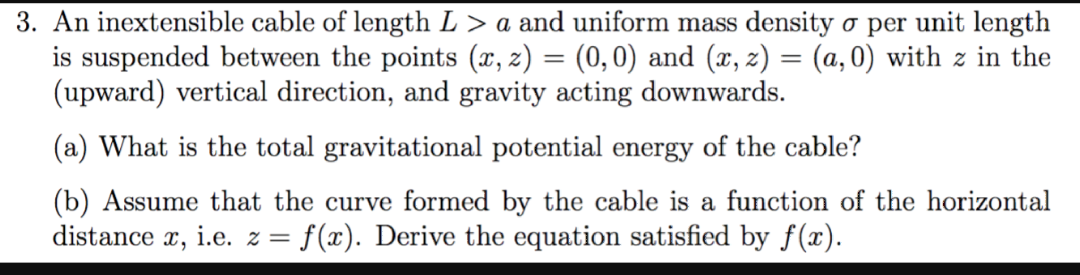# 3. An inextensible cable of length L > a and uniform mass density a per unit lengthis suspended between the points (x, 2) = (0,0) and (x, z) = (a, 0) with z in the(upward) vertical direction, and gravity acting downwards(a) What is the total gravitational potential energy of the cable?(b) Assume that the curve formed by the cable is a function of the horizontaldistance , ie. z = f(x). Derive the equation satisfied by f(x)

Questionhelp_outlineImage Transcriptionclose3. An inextensible cable of length L > a and uniform mass density a per unit length is suspended between the points (x, 2) = (0,0) and (x, z) = (a, 0) with z in the (upward) vertical direction, and gravity acting downwards (a) What is the total gravitational potential energy of the cable? (b) Assume that the curve formed by the cable is a function of the horizontal distance , ie. z = f(x). Derive the equation satisfied by f(x) fullscreen
check_circle

Step 1

a)

The gravitational potential energy is given by

Step 2

Here G is the gravitational constant, M is the mass of Earth, σ is the mass density R is the radius of the Earth and L is the length of the cable.

Step 3

b)

As the mass density is uniform, the curve can be semi circular.

The radius of the semi circ...

### Want to see the full answer?

See Solution

#### Want to see this answer and more?

Solutions are written by subject experts who are available 24/7. Questions are typically answered within 1 hour.*

See Solution
*Response times may vary by subject and question.
Tagged in

### Physics